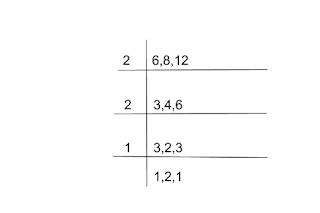# LCM And HCF Definition, Formula, Relation, Questions, Trick

In this article we explain LCM And HCF Definition, Formula, Relation between lcm and hcf, questions

### LCM And HCF Definition

#### LCM Definition ( Least Common Multiple )

The least common multiple of two or more numbers or expressions is the smallest number Or is an expression with a minimum of a square that is completely divisible by the given numbers or expressions.

Examples
Finding the least common multiple of 6, 8, 12 numbers.

The numbers divided by 6 are 6, 12, 18, 24, 30, 36

The numbers 8, 16, 24, 32, 40, divided by 8

The numbers 8, 16, 24, 32, 40, divided by 8

Numbers divided by 12 are 12, 24, 36

24 is the least common number among the numbers divided by these three numbers, so the least common multiple of these numbers is 24.

Similarly, the least common multiple of xЗ, x２, x５, x４ is x５.

Multiples of xЗ =, x４, x５, x６

Multiples of x２ = x２, xЗ, x４, x５, x６

Multiples of x５ = x５, x６, x７

Multiples of x４ = x４, x５, x６, x７

The common expression with the minimum address = x５. Thus, the least common multiple = x５

#### Method Of Finding LCM ( Least Common Multiple )

There are two methods to find the LCM .

#### Factor Method

In this method we first do a prime factorization of numbers.

Then we get the product of each factor of more powers.

For example, to find the least common multiple of 6,8,12, one must first do a prime factorization of these numbers.

Prime factorization of 6 =   2 × 3

Prime factorization of 12 = 2 × 2 × 3

Prime factorization of 8 =   2 × 2 × 2

Note – When we find the least common multiple, we include the common numbers as well as the other remaining numbers.

Explanation
Step 1 — The prime factor of 6,12,8 will include the common number 2. The following is the picture.

Step-2 Common will take 2 out of the prime factors of 8,12. In the following figure.

Step-3 Common will take 3 out of the prime factors of 6,12. In the following figure.

Step-4 Finally, the number 2 in the prime factor of 12 will also be included. In the following figure.

Thus, after following all the steps, the least common multiple of the number 6,8,12 = 2 x 2 x 3 x 2 = 24

Example-2
Find the least common multiple of xy, x２y, xy２?

Solve– The least common multiple of x 2 y = x❌x ❌y.

The least common multiple of x y2 = x❌y ❌y

The least common multiple of x y = x❌y

x y, x 2 y, the least common multiple of x y2 = x 2 y2
0000000000000
Explanation
Step-1 Common number in the factors of x y, x 2 y, x y2 = X . In the picture.

Step-2 Common number in the factors of x y, x 2 y, x y2 =Y . In the picture.

Step-3 At the end, the remaining XY will be included in the factors of x 2 y, x y2.

Step-4

### Division Method

In this method, the least common divisor is obtained by dividing by prime numbers.
Example
To find the least common multiple of 6, 8, 12 by this method.

##Minimum common factor of 6,8,12 = 2 x 2 x 3 x 1 x 2 x 1 = 24

### Highest Common Factor

#### Defination of Highest Common Factor

The largest number that completely divides each of the given numbers.The numbers are called the greatest common factor.
Example
Finding the maximum common factor of 6,8,12?
The numbers dividing 6 = 1,2,3,6.
The numbers dividing 8 = 1,2,4,8.
The number dividing 12 is = 1,2,3,4,6,12.
The maximum common number is 2 among the numbers dividing 8,14,12. It is clear that their maximum common factor is 2.

#### Method of finding HCF

1. Factor Method
In this method, the prime factors of the given numbers are derived.Then the product of all the rhinoceros is the greatest common factor.
Example
Finding the maximum common factor of 6,8,12?
6 = 2×8
8 = 2x2x2
12 = 2x2x2x3
than maximum common factor is 2
2. Division Method
In this method, the first is divided by the smallest number.

## 1 thought on “LCM And HCF Definition, Formula, Relation, Questions, Trick”

1.I

Today, I went to the beach with my children. I foud a sea shell and
gave iit to my 4 yewar old daughter and said “You can hear the ocean if you put this to your ear.”
She placed the shesll to her ear and screamed. There was
a hermit crab inside and it pinched her ear. She never wants to
go back! LoL I know this is entirely off topic but I